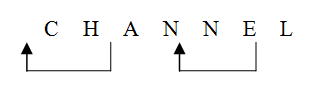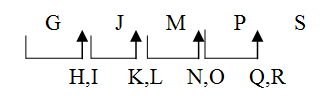# Tips, tricks and shortcuts in Alphabet Test : Learn Series

Dear Bankersdaily Aspirant,

Here we are posting our Second Article in Lear Series in Reasoning Section. The first article covers the concepts of Direction and Distance. This series surely helpful for the beginners who are preparing for Bank Exam and for others who already preparing can use this article for revising the Basic Concepts.

Introduction:

This article covers the topic of Alphabet Test questions based on which are asked under the reasoning section in various competitive exams like IBPS Clerk, SBI Clerk, SSC CGL, Placement Aptitude, IBPS PO, SBI PO, NICL AO, LIC  AAO, SBI Associate Clerk, SBI Associate PO, CAT and others.

Under this we deal with different types of questions based on English Alphabets, which could be asked in upcoming IBPS RRB, PO and Clerk.

## Important Facts of Alphabet Test:

1. Position number of letters in English2. Position number of letters in English alphabets in reverse3. We know A, E , I, O and U are vowels of English alphabet and remaining letters are consonants of English

4. A → 4 M (A to M) letters are called first half of English

5. N →  4 Z (N to Z) letters are called second half of English

6. To the left means Z →  A (Z to A)

7. To the right means A →  Z (A to Z)

8. From A →  Z (A to Z) letters are called left to right of English

9. From Z →  A (Z to A) letters are called right to left of English alphabets.

## Types of Question Based on Alphabet Test

There are four types of questions based on Alphabet test:

### Type- I Arrangement According To the Dictionary

Arranging words in alphabetical order implies ‘to arrange them in the order as they appear in a dictionary’. For this arrangement, first we shall consider the first letter of each word. Arrange the words in the order in which these letters comes in the English alphabets.

Example 1: The following four words are arranged in alphabetical order, which word will come in the second place

(a) Electric

(b) Elector

(c) Elect

(d) Electrode

Solution

Arranging the words in alphabetical order, we have : Elect, Elector, Electric, Electrode. Hence, Elector will come in the second place.

Example 2: Arrange the given words in alphabetical order and choose the one that comes at the third place?

(a) Bathing

(b) Banking

(c) Backing

(d) Banishing

Solution
Answer (d) Arrange the words in alphabetical order, we have, Backing, Banishing, Banking, Bathing.

Hence, Banking will come in the second place.

### Type 2 Alpha-Numeric Sequence

In these types of questions, a jumbled sequence of some letters, numbers and symbols are given. Sometimes only English alphabets from A to Z are given.

Example (Q. 3 to 7): Study the following arrangement carefully and answer the questions given below.Example 3: How many such symbols are there in the above arrangement, each of which is immediately preceded by a number and immediately followed by a letter?

(a) None

(b) One

(c) Two

(d) Three

Solution
Answer: (a) According to the question, in the given arrangement, none of such symbols is immediately preceded by a number and immediately followed by a letter.

Example 4: Three of the following four are alike in a certain way based on their positions in the above arrangement and so form a group. Which is the one that does not belong to that group?

(b) 58R

(c)  T V 8

(d) E%\$

Solution
Answer (d) Except ‘E % \$’all follow same rule in the given arrangements.

Example 5: If all the symbols in the above arrangement are dropped, which of the following will be the twelfth from the left end?

(a) 4

(b) K

(c) 2

(d) U

Solution

Answer (c) After droping all the symbols, the given arrangement order is

F 3 9 H A D I 4 E M K 2 U R P 5 W 8 1 T J V 7

­

Twelfth from the left

Example 6: How many such consonants are there in the above arrangement, each of which is immediately preceded by a number and not immediately followed by a symbol?

(a) None

(b) One

(c) Two

(d) Three

Solution
Answer: (c) According to the question, in the given arrangements two such consonants 1TJ and 5 W 8 are immediately preceded by a number and not immediately followed by a symbol.

### Type 3 Letter-Word Problems

In these types of problems, a certain word is given and candidates are required to answer asked, how many letters in the word is as far away from the beginning of the word as, it is from the beginning of the English alphabet. Sometimes, a certain word is given and then asked how many letters remain same in its position if they arrange in alphabetical order. Sometimes, a certain word is given and asked, if vowel and consonants constitutes

Example 8: How many such pairs of letters are there in the word CHANNEL each of which has as many

letters between them in the word as in the English alphabets?

(a) None

(b) One

(c) Two

(d) Three

SolutionSo, such number of pairs are: AC, LN

Example 9: How many such letters are there in the word PMCLNOB remain same in its position, if they are

arranged in alphabetical order?

(a) None

(b) One

(c) Two

(d) Three

Solution

P    M    C     L    N    O    B

B    C     L    M    N    O    P

So, such type of letters are N and O.

Example 10: Each vowel of the word GLADIOLUS is substituted with the next letter of the English

alphabetical series and each consonant is substituted with the letter preceding it. How many vowels are present

in the new arrangement?

(a) None

(b) One

(c) Two

(d) Three

Solution

G    L    A    D    I    O    L    U    S

F    K    B    C     J    P    K    V    R

So, none such vowels are present in the new arrangement.

### Type 4 Rule Detection

In these types of problems or questions, a certain rule is given in questions and candidates are asked. choose

the correct alternatives that follow this given rule.

Example 11: Find out the correct alternatives in which numbers of letters skipped in between adjacent letters in the series is two?

(a) LORTW

(b) GJMPS

(c) KNPSV

(d) EHKLO

SolutionArticle Courtesy:

Mr. Nareshkumar

Reasoning Trainer

RACE Institute